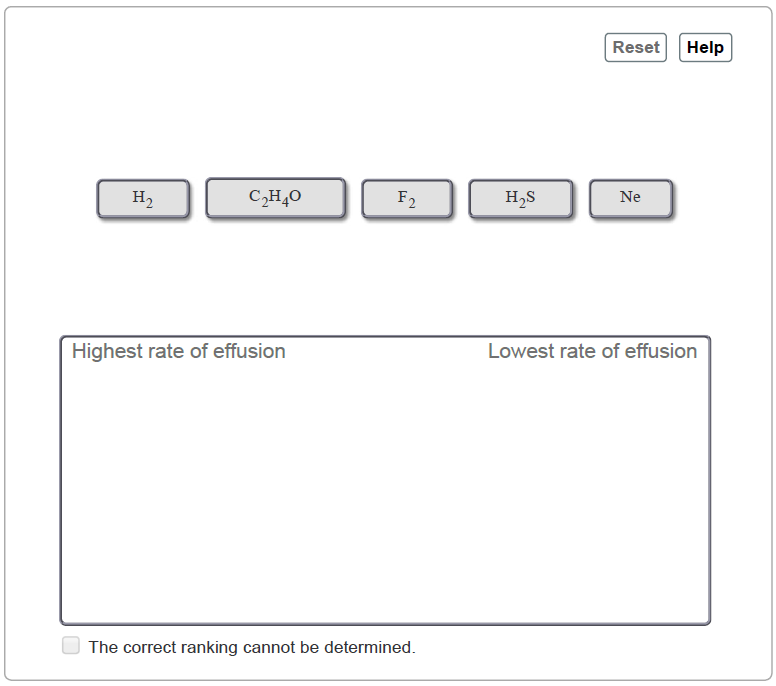# Problem: Rank the following gases in order of decreasing rate of effusion.Rank from the highest to lowest effusion rate. To rank items as equivalent, overlap them.In a given sample of gas, the particles move at varying speeds. The root mean square speed (rms speed) of particles in a gas sample, u, is given by the formulau=3RTMwhere T is the Kelvin temperature, M is the molar mass in kg/mol, and R = 8.314 J/(mol ⋅ K) is the gas constant. Effusion is the escape of gas molecules through a tiny hole into a vacuum.The rate of effusion of a gas is directly related to the rms speed of the gas molecules, so it's inversely proportional to the square root of its mass. The rms speed is related to kinetic energy, rather than average speed, and is the speed of a molecule possessing a kinetic energy identical to the average kinetic energy of the sample.Given its relationship to the mass of the molecule, you can conclude that the lighter the molecules of the gas, the more rapidly it effuses. Mathematically, this can be expressed aseffusion rate∝1mThe relative rate of effusion can be expressed in terms of molecular masses mA and mB asrate of gas A effusionrate of gas B effusion=mBmA

###### FREE Expert Solution
84% (318 ratings)View Complete Written Solution
###### Problem Details

Rank the following gases in order of decreasing rate of effusion.

Rank from the highest to lowest effusion rate. To rank items as equivalent, overlap them.In a given sample of gas, the particles move at varying speeds. The root mean square speed (rms speed) of particles in a gas sample, u, is given by the formula

$\mathbf{u}\mathbf{=}\sqrt{\frac{\mathbf{3}\mathbf{RT}}{\mathbf{M}}}$

where T is the Kelvin temperature, M is the molar mass in kg/mol, and R = 8.314 J/(mol ⋅ K) is the gas constant. Effusion is the escape of gas molecules through a tiny hole into a vacuum.

The rate of effusion of a gas is directly related to the rms speed of the gas molecules, so it's inversely proportional to the square root of its mass. The rms speed is related to kinetic energy, rather than average speed, and is the speed of a molecule possessing a kinetic energy identical to the average kinetic energy of the sample.

Given its relationship to the mass of the molecule, you can conclude that the lighter the molecules of the gas, the more rapidly it effuses. Mathematically, this can be expressed as

The relative rate of effusion can be expressed in terms of molecular masses mA and mB as